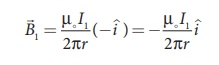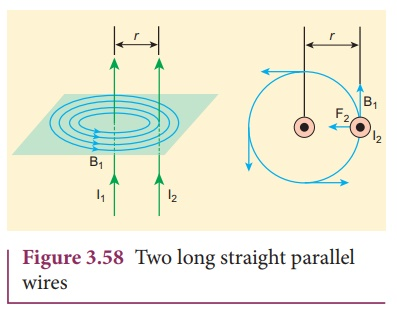Home | | Physics 12th Std | Force between two long parallel current carrying conductors

# Force between two long parallel current carrying conductors

Physics : Magnetism and Magnetic Effects of Electric Current: Lorentz Force: Force between two long parallel current carrying conductors

Force between two long parallel current carrying conductors

Two long straight parallel current carrying conductors separated by a distance r are kept in air as shown in Figure 3.58. Let I1 and I2 be the electric currents passing through the conductors A and B in same direction (i.e. along z - direction) respectively. The net magnetic field at a distance r due to current I1 in conductor A isFrom thumb rule, the direction of magnetic field is perpendicular to the plane of the paper and inwards (arrow into the page ) i.e. along negative i ^ direction.

Let us consider a small elemental length dl in conductor B at which the magnetic field B1 is present. From equation 3.65, Lorentz force on the element dl of conductor B isTherefore the force on dl of the wire B is directed towards the wire W1. So the length dl is attracted towards the conductor A. The force per unit length of the conductor B due to the wire conductor A isIn the same manner, we compute the magnitude of net magnetic induction due to current I2 (in conductor A) at a distance r in the elemental length dl of conductor A isFrom the thumb rule, direction of magnetic field is perpendicular to the plane of the paper and outwards (arrow out of the page ʘ) i.e., along positive i ^ direction.

Hence, the magnetic force at element dl of the wire is W1 isTherefore the force on dl of conductor A is directed towards the conductor B. So the length dl is attracted towards the conductor B as shown in Figure (3.59).The force per unit length of the conductor A due to the conductor B isThus the force experienced by two parallel current carrying conductors is attractive if the direction of electric current passing through them is same as shown in Figure 3.60.Thus the force experienced by two parallel current carrying conductors is repulsive if they carry current in the opposite directions as shown in Figure 3.61.

## Definition of ampère

One ampère is defined as that current when it is passed through each of the two infinitely long parallel straight conductors kept at a distance of one meter apart in vacuum causes each conductor to experience a force of 2 × 10−7 newton per meter length of conductor.

Tags : Lorentz Force | Physics , 12th Physics : Magnetism and Magnetic Effects of Electric Current
Study Material, Lecturing Notes, Assignment, Reference, Wiki description explanation, brief detail
12th Physics : Magnetism and Magnetic Effects of Electric Current : Force between two long parallel current carrying conductors | Lorentz Force | Physics